Question

(a) Find the equivalent resistance between points a and b in Figure P18.2. (b) Calculate the current in each resistor if a potential difference of 34.0 V is applied between points a and b.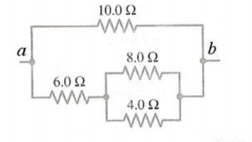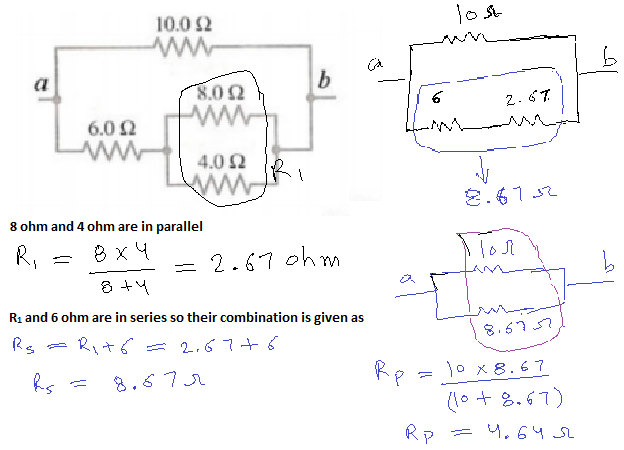so equivalent resistance, Rtotal = 4.64 ohm

b)

Total current at point A = itotal = V/Rtotal

itotal = (34)/(4.64) = 7.33 A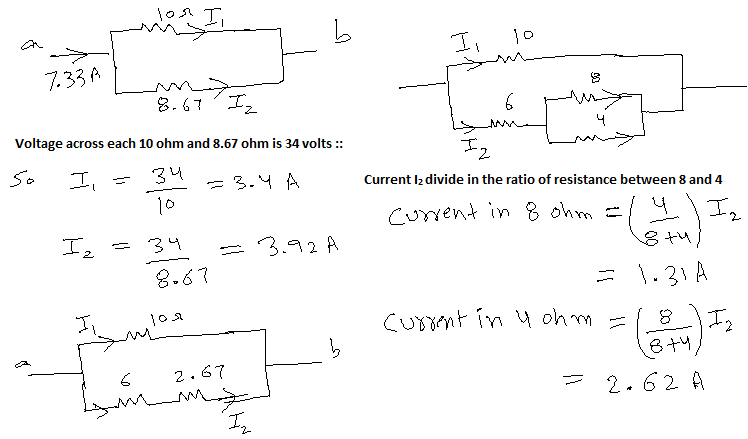#### Earn Coins

Coins can be redeemed for fabulous gifts.

Similar Homework Help Questions
• ### (a) Find the equivalent resistance between points a and b in the figure below (R = 17.0.2).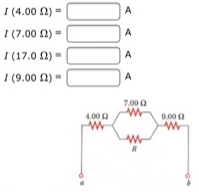(a) Find the equivalent resistance between points a and b in the figure below (R = 17.0.2). (b) A potential difference of 34.0 V is applied between points a and b. Calculate the current in each resistor.

• ### (a) Find the equivalent resistance between points a and b in the figure. (R = 17.0...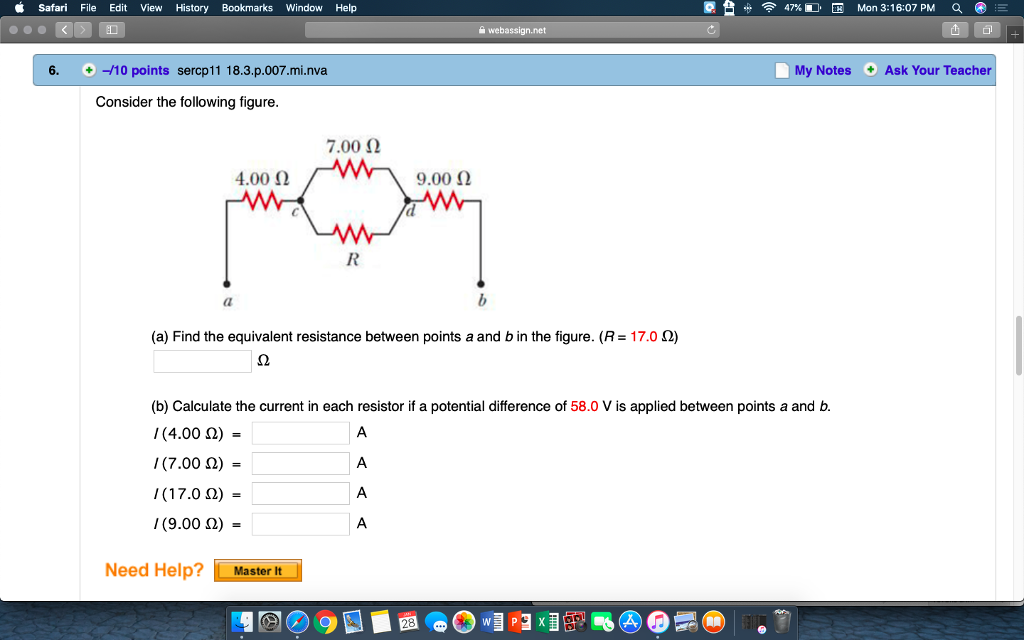(a) Find the equivalent resistance between points a and b in the figure. (R = 17.0 Ω) (b) Calculate the current in each resistor if a potential difference of 58.0 V is applied between points a and b. I (4.00 Ω) =   A I (7.00 Ω) =   A I (17.0 Ω) =   A I (9.00 Ω) =   A Please answer all parts to A and B correctly!!!! Thank you so much in advance! Safari File Edit View History Bookmarks Window...

• ### (a) Find the equivalent resistance between points a and b in the figure. (R=20.0Ω)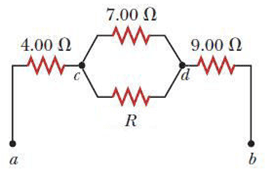(a) Find the equivalent resistance between points a and b in the figure. (R=20.0Ω) (b) Calculate the current in each resistor if a potential difference of 40.0 V is applied between points a and b.

• ### a) Find the equivalent resistance between points a and b in Figure 1 (R = 17.00 22). If a potential difference of...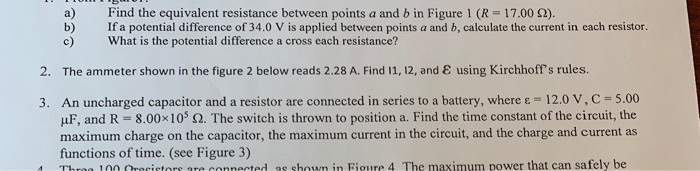a) Find the equivalent resistance between points a and b in Figure 1 (R = 17.00 22). If a potential difference of 340 V is applied between points a and b, calculate the current in each resistor. What is the potential difference a cross each resistance? 2. The ammeter shown in the figure 2 below reads 2.28 A. Find 11, 12, and using Kirchhoff's rules. 3. An uncharged capacitor and a resistor are connected in series to a battery, where...

• ### Practice Another Version BerCP PO (a) Find the equivalent resistance between points a and b in...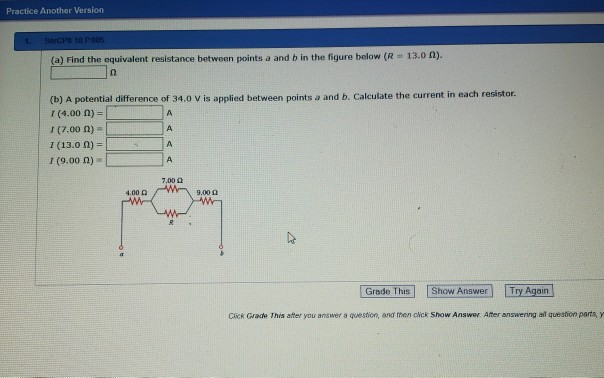Practice Another Version BerCP PO (a) Find the equivalent resistance between points a and b in the figure below ( R 13.0 ). (b) A potential difference of 34.0 Vis applied between points a and b. Calculate the current in each resistor 1(4.00) (7.00 A) - (13.0 A) = 1 (9.00 n) - Grade This Show Answer Try Again Cik Grace This atter you answer question, and then click Show Answer After answering all question party

• ### Example 1: Four resistors are connected as shown (A) Find the equivalent resistance between points a...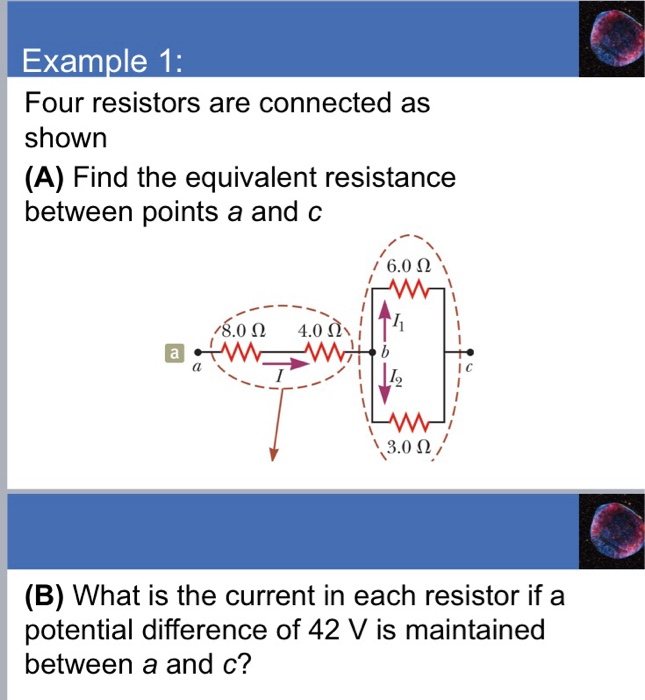Example 1: Four resistors are connected as shown (A) Find the equivalent resistance between points a and c /80 Ω 4.0(). IIT, I C 9 3,0 Ω (B) What is the current in each resistor if a potential difference of 42 V is maintained between a and c?

• ### a) Find the equivalent resistance between the points A and C in the wing. (b) If...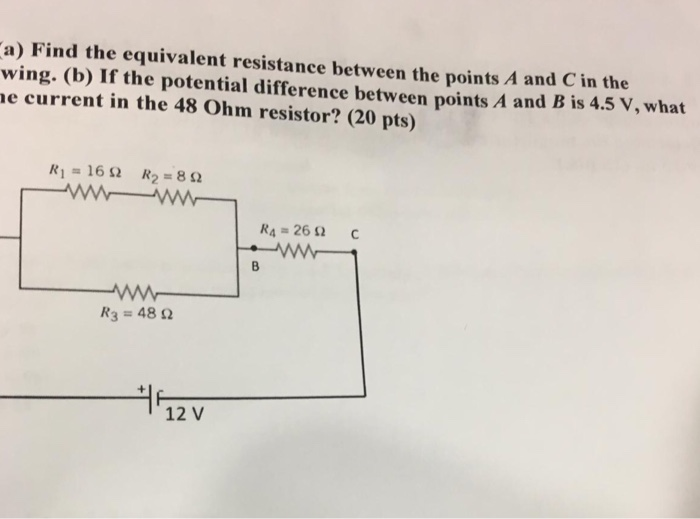a) Find the equivalent resistance between the points A and C in the wing. (b) If the potential difference between points A and B is 4.5 V, what e current in the 48 Ohm resistor? (20 pts) Ri=16Ω R2 = 8 Ω R4-26 Ω R3 = 48 Ω 12 V

• ### Consider the following figure 7.00 Ω 4.00 Ω 9.00 Ω 15.0 Ω) (a) Find the equivalent...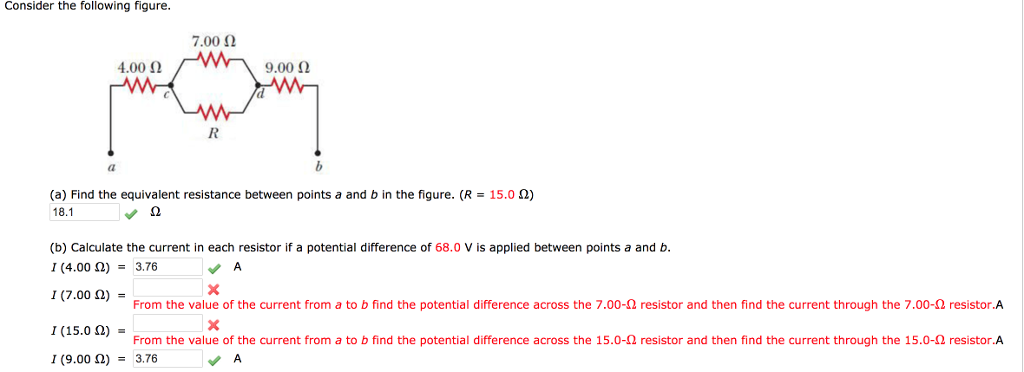Consider the following figure 7.00 Ω 4.00 Ω 9.00 Ω 15.0 Ω) (a) Find the equivalent resistance between points a and b in the figure. (R 18.1 (b) Calculate the current in each resistor if a potential difference of 68.0 V is applied between points a and b 1 (4.00 Ω)-3.76 、A 1 (7.00A)the From the value of the current from a to b find the potential difference across the 7.00 Ω res stor and the find the current throug...

• ### (a) Find the equivalent resistance between point a and b. (b) If a voltage of 62...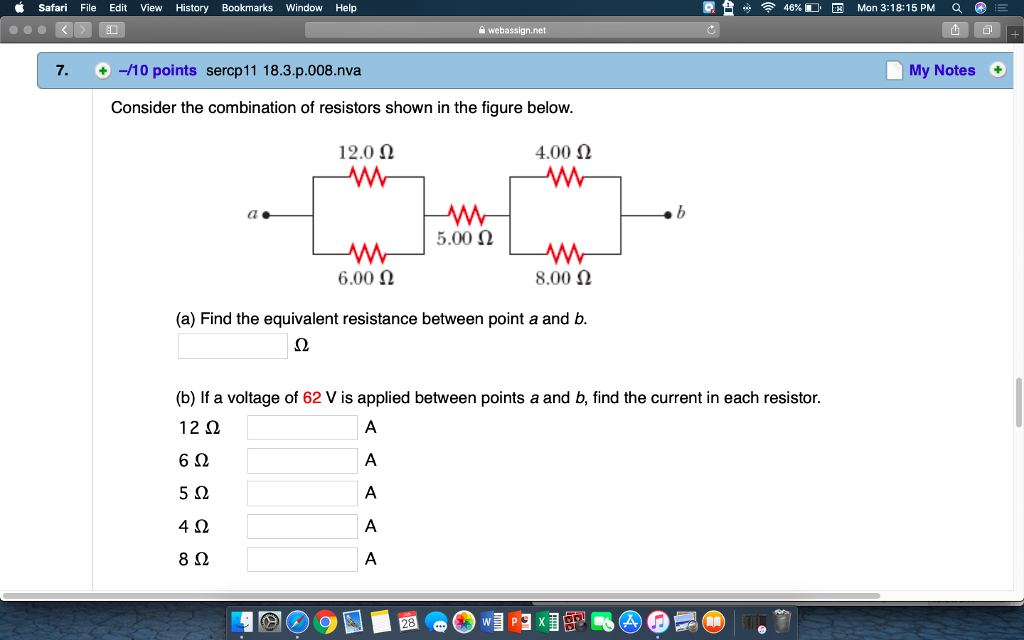(a) Find the equivalent resistance between point a and b. (b) If a voltage of 62 V is applied between points a and b, find the current in each resistor. Please answer all parts to A and B correctly!!!! Thank you so much in advance! Safari File Edit View History Bookmarks Window Help 46% Mon 3:18:15PM 'Q 7. +-/10 points sercp11 18.3.p.008.nva My Notes Consider the combination of resistors shown in the figure below. 12.0 Ω 4.00 Ω ao AM...